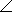# Electrical Engineering - RC Circuits - Discussion

### Discussion :: RC Circuits - General Questions (Q.No.22)

22.

The complex number 4055° is equivalent to

 [A]. 55 + j55 [B]. 40 + j40 [C]. 45.88 + j65.52 [D]. 22.94 + j32.76

Explanation:

No answer description available for this question.

 Dilip Verma said: (Sep 7, 2013) z=root(a2+b2) where z=40 and Angle=a/b where angle=tan55 then by making equations we solve it.

 Nihar said: (Sep 24, 2013) A